# Improving the Results and Efficiency of My Options Trading GA

In a previous post, I wrote about a genetic algorithm (GA) whose goal is to find stocks that could potentially create a promising put-selling portfolio. Currently, the GA searches through stocks that offer weekly options and tries to build a portfolio with three objectives:

1. Maximize the premium earned when selling the options
2. Minimize the risk, which in this case is stock price volatility
3. Build a portfolio for which the user has enough cash collateral to actually trade

Although I don’t use the algorithm’s results directly to sell puts, it has become an interesting resource for providing ideas on which stocks might show promise in a put-selling (or wheel) portfolio. Running the algorithm and properly analyzing the stocks it suggests can provide good additions to an option selling portfolio.

However, after running the algorithm quite a few times in the last month or so, a number of problems have arisen. First and foremost, the GA typically doesn’t use all of the portfolio’s available collateral. This leads to ineffective use of capital and a poorly diversified portfolio. A second issue is that the algorithm takes a significant amount of time to run using the Python implementation. The third and final issue I have addressed is the distribution of the algorithm (although I won’t share it with everyone). I have had close friends and family express interest in running the algorithm but have had difficulty setting up the Python environment and modifying the algorithm to suit their needs. Unfortunately, I don’t think I’m able to share the data I’m gathering to run the algorithm with a large audience so I will not be able to share the web address of the interface I’ve implemented to make the algorithm easier to run. I will share the implementation details though.

## A Re-Evaluated Objective Function

In the previous Python implementation of the algorithm, the objective function considered three things: i) the average distance of the beta value from 0 for each stock, ii) the amount of income (premium) earned from wheeling the stock, and iii) the usage of collateral. More detailed information can be found in my previous post. In effect, the fitness of an individual in the population is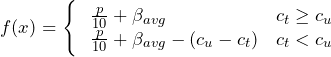Where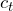is the available collateral in the portfolio and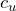is the collateral used by the individual. The fitness is adjusted downwards if the individual uses too much collateral but nothing is done if the individual uses too little collateral. The adjustment effectively weeds out portfolios that use too much collateral as the typical fitness value is between 0 and 4, due to the weighting, and the collateral adjustment takes it much further than that into negative value territory.

The lack of adjustment for using too little collateral is the main issue with the objective function however, two other adjustments were made to the objective function as well. The first uses an updated measurement of volatility (therefore risk, in this case) and the second includes the number of individual tickers in the portfolio with a small preference being given to portfolios with many holdings for diversification’s sake.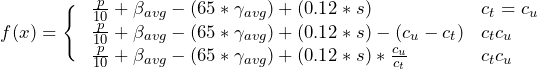where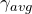is the average daily percent change of the stock price which is used as another measurement of volatility,andare defined above, s is the number of tickers in the portfolio, and p is the premium earned from selling an automatically selected put option for the stock. It should be noted that the weights used on these variables are configured due to their size with respect to the other variables and their importance in the overall portfolio.

As seen here the new objectives are incorporated in the fitness function. The s variable affords the individuals a small preference to portfolios with more holdings, and the score is now reduced to a fraction of its former value if it does not use all of the available collateral.

When running the GA for a reasonable number of generations (1,000+) and population size of 5,000 individuals, I’ve yet to see a portfolio with under 80% of its collateral being used. Typically the algorithm generates a portfolio using 95%+ of the available collateral and one which returns a pretty reasonable risk/reward metric, in my opinion (this is obviously highly subjective).

## A Partial Re-Implementation in C++ With Data Caching

The second enhancement to the algorithm overall was to reimplement the GA portion in C++ using GALib. This implementation runs quite a bit faster than the Python version, which is really the only upside. The full code can be found at the end of the post but I will omit it here as to not hide the rest of the content.

Only the GA implementation was re-written in C++, all other functionality (data fetching) was left in Python. This was because I had built a substantial amount of tooling to be able to write this portion of the algorithm in Python via my Ally Invest API wrapper (this has been updated since the post was published), which I didn’t want to have to redo in C++. The Python script was modified to output the option data to a CSV file which can then be read using C++ DataTables which I also wrote about in a previous post.

A cron job was set up to run the data fetching script every 30 minutes which updates the current strike prices, premiums, and beta measurements. Because of this, it is best to run the algorithm after hours when the prices don’t fluctuate, or approx. 3-5 minutes after every 30 minute or hour mark during normal trading hours when the data is most up-to-date. Caching the data in this way can lead to problems as the data becomes outdated but drastically decreases the runtime of the algorithm due to Ally Invest’s API request limits and the large number of companies that data is being fetched for (~500).

## Using Node.js and Express to Serve the Application

The third and final enhancement was a node.js/express GET request which can be called to run the algorithm. The process to run the GA is spawned in JavaScript with the parameters specified in the GET URL. This process then outputs a JSON file containing the metadata used to run the algorithm and the resulting portfolio. This JSON file is later read by a Python emailing script which sends an email of the results. This script is also scheduled to run via cron every 5 minutes.

The code for the node.js is presented in its entirety below.

const execFile = require('child_process').execFile;
var express = require('express');
const util = require('util');
var app = express();

app.get('/garequest', (req, res) => {
if(req.query.collateral === undefined || req.query.email === undefined || req.query.generations === undefined) {
return res.send("Bad parameters: collateral, generations, and email are required.");
}

var command = "optimize"; // the C++ executable name
var params = [req.query.collateral, req.query.generations, req.query.email];
const proc = execFile(command, params);

console.log(command);
console.log(params);

let response = 'Started GA process, your results will be emailed to you.';
res.status(200).send(response);
});

var port = 9009;
app.listen(port, () => console.log("Started GA API on port " + port))

This server is continuously running via forever. I’d like to share the URL so that anyone could run the GA and get emailed the results but, unfortunately, I don’t think I’m able to “distribute” the data from Ally Invest in that way. Anyone interested in building their own implementation can get an Ally Invest account and API keys and use the scripts in this post and the previous post on the topic to create their own implementation though. The full code for this version is given below.

## Conclusion

In this post, I outline some recent enhancements to my GA used to generate put-selling portfolios. The enhancements discussed here have improved the performance of the algorithm due to the updated fitness function, have improved its runtime which provides results in a more timely manner, and have made the algorithm more accessible to those without a programming background.

Anyone interested in trading options in this way should be sure to do their research to fully understand the risks being taken and should not solely rely on projects like this to do their research for them.

## Full Code

#### server.js

const execFile = require('child_process').execFile;
var express = require('express');
const util = require('util');
var app = express();

app.get('/garequest', (req, res) => {
if(req.query.collateral === undefined || req.query.email === undefined || req.query.generations === undefined) {
return res.send("Bad parameters: collateral, generations, and email are required.");
}

var command = "optimize"; // the C++ executable name
var params = [req.query.collateral, req.query.generations, req.query.email];
const proc = execFile(command, params);

console.log(command);
console.log(params);

let response = 'Started GA process, your results will be emailed to you.';
res.status(200).send(response);
});

var port = 9009;
app.listen(port, () => console.log("Started GA API on port " + port))

#### emailer.py

import smtplib
from email.mime.text import MIMEText
import os
import json

basedir = '<directory_to_this_script>'
outdir = '/archive/'

def build_msg(jdata):
msgTxt = "Number of Generations: " + str(jdata["numberGenerations"]) + "\n"
msgTxt += "Collateral Submitted: " + str(jdata["submittedCollateral"]) + "\n"
msgTxt += "Collateral % Used: " + jdata["collateralPctUsage"] + "\n"
msgTxt += "Number of Tickers in Portfolio: " + str(jdata["numberTickers"]) + "\n"
msgTxt += "Total Collateral: " + str(jdata["totalCollateral"]) + "\n"
msgTxt += "Total Income: " + str(jdata["totalIncome"]) + "\n"
msgTxt += "Average Beta: " + str(jdata["avgBeta"]) + "\n"
msgTxt += "Portfolio Avg Daily Pct Change: " + jdata["portfolioAvgPctChange"] + "\n"
msgTxt += "Percent Income: " + jdata["percentIncome"] + "\n"

msgTxt += "\nPortfolio:\n"
for item in jdata['portfolio']:
msgTxt += "\tSymbol: " + item['symbol'] + "\n"
msgTxt += "\tIncome: " + str(item['income']) + "\n"
msgTxt += "\tStrike Price: " + str(item['strike']) + "\n"
msgTxt += "\tBeta: " + str(item['beta']) + "\n"
msgTxt += "\tAverage Daily Percent Change: " + item['avgDailyPctChange'] + "\n"
msgTxt += "\tExpiration: " + item['expirationDate'] + "\n"
msgTxt += "\n"

msg = MIMEText(msgTxt)
msg['Subject'] = "GA Results"
msg['From'] = "your_email@gmail.com"
msg['To'] = jdata['email']

return msg

try:
server = smtplib.SMTP('smtp.gmail.com', 587)
server.ehlo()
server.starttls()
server.quit()
except:
outdir += 'ERROR.'
print("Something went wrong")

for f in os.listdir(basedir):
outdir = '/archive/'
if f.endswith('.results'):
try:
handle = open(basedir + f)
msg = build_msg(data)
send_email(msg, data['email'])
except:
outdir += '' if outdir.endswith('ERROR.') else 'ERROR.'
finally:
os.rename(basedir + f, basedir + outdir + f)

#### data_fetcher.py

import pandas as pd
from ally import AllyAPI
from ally.requests import QuotesRequest
import datetime
import time
import json
import os

class DataFetcher(object):
def __init__(self, ticker_filename, collateral,  expiration_date = None,
consumer_key="your_ally_invest_key",
oauth_token="your_ally_invest_key",
oauth_secret="your_ally_invest_key",
strikes_out=1):
"""
ticker_filename -> filename for tickers to be considered (csv)
expiration_date -> expiration date of the options considered
collateral -> amount of funds available as collateral
strikes_out -> how many strikes below current price
- note that this is only for Puts for Calls options are
"""
self.filename = ticker_filename

self.strikes_out = strikes_out
self.collateral = collateral

self.CONSUMER_KEY = consumer_key
self.OAUTH_TOKEN = oauth_token
self.OAUTH_SECRET = oauth_secret

self.ally = AllyAPI(self.OAUTH_SECRET, self.OAUTH_TOKEN, self.CONSUMER_KEY, response_format='json')
self.expiration = self.__infer_next_expiration() if expiration_date is None else expiration_date

def fetch_data(self):

# get stock quotes for all tickers; keep price, symbol, and beta measurement
data = []
tickers_per_request = 400
tickers = ticker_csv["Ticker"].tolist()
tickers = [tickers[x:x+tickers_per_request] for x in range(0, len(tickers), tickers_per_request)]
for ticker_list in tickers:
quote_request = QuotesRequest(symbols=ticker_list)
response = quote_request.execute(self.ally)
for quote in response.get_quotes():
# bad fetch or too expensive or bankrupt
if quote.symbol == 'na' or float(quote.last)*100 > self.collateral \
or float(quote.bid) == 0 or float(quote.ask) == 0 or quote.beta == '':
continue

# get available strike prices for all tickers (ally.get_options_strikes(symbol))
count = 0
for dp in data:
if count == 100:    # this isn't the rate limit but there are other processes using the API
print("Rate limit reached, sleeping for 15 seconds...")
time.sleep(15)
count = 0
js = self.ally.get_options_strikes(dp)   # unfortunately, this can't take multiple tickers
strikes = js['response']['prices']['price']
# get index of nearest strike less than current price
idx = next((x for x, val in enumerate(strikes) if float(val) > dp), 0) - self.strikes_out
if idx < 0:
dp.append(0)
continue
strike_price = float(strikes[idx])
dp.append(strike_price)
count += 1

tickers_per_request = 100
tickers = ticker_csv["Ticker"].tolist()
tickers = [tickers[x:x+tickers_per_request] for x in range(0, len(tickers), tickers_per_request)]
for ticker_list in tickers:
putcall, dates = [], []
for _ in range(len(ticker_list)):
putcall.append("p")
dates.append(self.expiration)

tickers, strikes = [], []
for ticker in ticker_list:
for dp in data:
if dp == ticker:
tickers.append(ticker)
strikes.append(dp)
break

quote = self.ally.get_option_quote(tickers, dates, strikes, putcall)['response']['quotes']['quote']
for q in quote:
for dp in data:
if dp == q['undersymbol']:
break

self.expiration = self.expiration.strftime("%Y-%m-%d")
for dp in data:
dp.append(self.expiration)

return pd.DataFrame(data, columns=['symbol', 'price_mid', 'beta', 'option_strike', 'option_income', 'expiration'])

def fetch_to_csv(self, filename='option_data.csv'):
df = self.fetch_data()
df = df.dropna()
jdata = df.to_csv(filename, index=False)

def __infer_next_expiration(self):
js = self.ally.get_options_expirations("aapl")
count = 0
while count < len(js["response"]["expirationdates"]['date']):
today = datetime.datetime.now()
js_date = datetime.datetime.strptime(js["response"]["expirationdates"]['date'][count], "%Y-%m-%d")
if (js_date - today).days >= 6:
return js_date
count += 1
return datetime.datetime(1991, 12, 31)

if __name__ == '__main__':
fetcher = DataFetcher('weekly_option_tickers.csv', 250000, strikes_out=1)
fetcher.fetch_to_csv(filename="cached_option_data.csv")

#### optimize.cpp

#include <iostream>
#include <time.h>
#include <string>
#include <fstream>
#include <exception>
#include <sstream>
#include <vector>
#include <ga/ga.h>
#include <math.h>
#include <chrono>
#include <random>
#include <DataTable/DataTable.hpp>
#include <map>

#include "security.hpp"
#include "parameters.hpp"
#include "yfapi.hpp"

Parameters parameters;
std::map<std::string, datatable::DataTable<float>> tickerToMonthlyDataMap;

struct OptimizeException : public std::runtime_error
{
OptimizeException(std::string msg) : runtime_error(msg) {}
};

std::vector<Security> getSecuritiesFromFile(std::string filename)
{
int cols = 6;
std::ifstream data_file(filename);
if(!data_file.is_open())
throw OptimizeException("ERROR: Unable to open file '" + filename + "', no data has been loaded.");

std::vector<std::string> lines;
std::string line;
while(std::getline(data_file, line))
lines.push_back(line);

std::vector<Security> securities;
for(auto& line : lines)
{
int col_count = 0;
std::string value;
std::istringstream ss2(line);

Security security;
while(std::getline(ss2, value, ','))
{
if(value == "NVAX") break;		// TODO: remove later? inaccurate beta
switch (col_count)
{
case 0:
security.symbol = value;
break;
case 1:
security.priceMid = std::stof(value);
break;
case 2:
security.beta = std::stof(value);
break;
case 3:
security.optionStrike = std::stof(value);
break;
case 4:
security.optionIncome = std::stof(value);
break;
case 5:
security.expiration = value;
break;
}
col_count++;
}

// calcuate avg daily pct change
try
{
datatable::DataTable<float> dt(parameters.basedir + "cache/" + security.symbol + ".csv", "Close");
float* pctChange = dt.pct_change("Close");
float avgPctChange = 0.0;
for(int i = 0; i < dt.nrows() - 1; i++)
avgPctChange += abs(pctChange[i]);
security.avgDailyPctChange = avgPctChange / ((float)dt.nrows()-1.0);
}
catch(...)
{
security.avgDailyPctChange = 100.0;		// set very high, don't consider the stock if we don't have complete information
}

securities.push_back(security);
}

return securities;
}

float Objective(GAGenome& genome)
{
GA1DBinaryStringGenome &g = (GA1DBinaryStringGenome &) genome;
yfapi::YahooFinanceAPI api;
float score = 0.0, usedColl = 0.0, income = 0.0, beta = 0.0, avgPctChange = 0.0;

int count = 0;
for(int i = 0; i < g.length(); i++)
{
if(g.gene(i) == 1)
{
Security sec = parameters.securities.at(i);
usedColl += sec.optionStrike*100;
beta += (1 - abs(sec.beta*2));		// *X since this seems to be way too low for most tickers (compare to YahooFinance)
income += sec.optionIncome;
avgPctChange += sec.avgDailyPctChange;

count++;
}
}

beta = count == 0 ? beta : beta/(float)count;
avgPctChange = count == 0 ? avgPctChange : avgPctChange / (float)count;
score = 0.1*income + beta - 65.0*avgPctChange;
score += (.12*(float)count);	// small preference to more holdings

score -= usedColl > parameters.collateral ? (usedColl - parameters.collateral) : 0;
score *= usedColl < parameters.collateral ? (float)((float)usedColl/(float)parameters.collateral) : 1.0;

return score;
}

void MostlyZeroInitializer(GAGenome &genome)
{
GA1DBinaryStringGenome &g = (GA1DBinaryStringGenome &) genome;
std::mt19937_64 rng;
uint64_t timeSeed = std::chrono::high_resolution_clock::now().time_since_epoch().count();
std::seed_seq ss{uint32_t(timeSeed & 0xffffffff), uint32_t(timeSeed>>32)};
rng.seed(ss);

std::uniform_real_distribution<double> unif(0, 1);

for (int i = 0; i < g.length(); i++)
{
double currentRandomNumber = unif(rng);
// 9x% chance of being a 0, y% chance of being a 1
g.gene(i, currentRandomNumber < .97 ? 0 : 1);
}
}

int main(int argc, char* argv[])
{
try
{
parameters.parse(argc, argv);
}
catch(ParameterParseException& p)
{
throw p;
// write exception to file and email requester
}

// fetch and read cached option data

std::string filename(parameters.basedir + "cached_option_data.csv"); // get last mod time, add to file output
parameters.securities = getSecuritiesFromFile(filename);

GA1DBinaryStringGenome genome(parameters.securities.size(), Objective);
genome.initializer(MostlyZeroInitializer);
GASigmaTruncationScaling scaling;

GASimpleGA ga(genome);
ga.populationSize(5000);
ga.nGenerations(parameters.generations);
ga.pMutation(0.001);
ga.pCrossover(1);
ga.scaling(scaling);
ga.flushFrequency(100);

ga.evolve();

auto now = std::chrono::system_clock::now();
auto epoch = now.time_since_epoch();
auto ms = std::chrono::duration_cast<std::chrono::microseconds>(epoch);

std::string outputFilename = parameters.basedir + std::to_string(ms.count()) + parameters.email + ".results";
std::ofstream outputFile(outputFilename);
if(!outputFile.is_open())
throw OptimizeException("Cannot open output file."); 	// log somehow

outputFile << "{" << std::endl;
outputFile << "\t\"email\": \"" << parameters.email << "\", " << std::endl;
outputFile << "\t\"portfolio\" : [" << std::endl;

GA1DBinaryStringGenome &g = (GA1DBinaryStringGenome &)ga.statistics().bestIndividual();
float totalIncome = 0.0, totalCollateral = 0.0, avgBeta = 0.0, portfolioAvgPctChange = 0.0;
int numTickers = 0;
std::string outputString;
for(int i = 0; i < g.length(); i++)
{
if(g.gene(i) == 1)
{
outputString += "\t\t{\n";
Security sec = parameters.securities.at(i);

numTickers++;
totalIncome += sec.optionIncome;
totalCollateral += sec.optionStrike*100.0;
avgBeta += (sec.beta);
portfolioAvgPctChange += sec.avgDailyPctChange;

outputString += "\t\t\t\"symbol\": \"" + sec.symbol + "\", \n";
outputString += "\t\t\t\"income\": " + std::to_string(sec.optionIncome*100) + ", \n";
outputString += "\t\t\t\"strike\": " + std::to_string(sec.optionStrike) + ", \n";
outputString += "\t\t\t\"beta\": " + std::to_string(sec.beta) + ", \n";
outputString += "\t\t\t\"avgDailyPctChange\": \"" + std::to_string(sec.avgDailyPctChange*100) + "%\", \n";
outputString += "\t\t\t\"expirationDate\": \"" + sec.expiration + "\"\n";
outputString += "\t\t},\n";
}
}
totalIncome *= 100;

if(outputString.length() > 0)
outputString = outputString.substr(0, outputString.length() - 2);
outputFile << outputString << std::endl;

outputFile << "\t]," << std::endl;
outputFile << "\t\"submittedCollateral\": " << parameters.collateral << ", " << std::endl;
outputFile << "\t\"collateralPctUsage\": \"" << (100.0*((float)totalCollateral/(float)parameters.collateral)) << "%\", " << std::endl;
outputFile << "\t\"numberGenerations\": " << parameters.generations << ", " << std::endl;
outputFile << "\t\"numberTickers\": " << numTickers << ", " << std::endl;
outputFile << "\t\"totalIncome\": " << (totalIncome) << ", " << std::endl;
outputFile << "\t\"totalCollateral\": " << totalCollateral << ", " << std::endl;
outputFile << "\t\"avgBeta\": " << (avgBeta/(float)numTickers) << ", " << std::endl;
outputFile << "\t\"portfolioAvgPctChange\": \"" << (100*(portfolioAvgPctChange/(float)numTickers)) << "%\", " << std::endl;
outputFile << "\t\"percentIncome\": \"" << (100.0*(totalIncome/totalCollateral)) << "%\"" << std::endl;

outputFile << "}";
outputFile.close();

return 0;
}